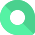# Net Present Value

## What is Net Present Value?

Net Present Value is the difference between the present value of cash inflows and the present value of cash outflows, over a period of time. It is used to determine the viability of an initiative or investment.

### How to calculate Net Present Value

ƒ -(Invested Cash) + ((Net Cash during a first period) / (1 + Discount Rate or Alternative Rate of Return during first period)) + ((Net Cash during n period) / (1 + Discount Rate or Alternative Rate of Return during n period to the power of n))

### What is a good Net Present Value benchmark?

An investment is viable if the NPV is positive.

### Example

Company A has a 7.5 year interest-free, long-term loan of \$500,000. The current bank loan rate of 5% and monthly payment required of \$8,333 will start on the 31st month. To calculate the current value of the loan: In the Excel NPV tool: Rate: 5%/12=0.42%, Value1-value 30:\$0, Value31-Value 89 : \$8,333, CPT NPV=\$389,800.50. This means the company will spend the cost at the present value of \$389,800.50 to obtain the loan of \$500,000.

The NPV rule states that companies must only invest in projects that have a positive NPV. Intuitively, this rule implies that it is only worth investing in projects where the return exceeds the cost of investment.

The amount of money generated by future cash flows, less the actual cost of investing in these cash flows is the NPV of the project or investment. The process of computing the present value of a future cash flow is called discounting and this is essential for calculating the worth of the future return of the project in today’s terms. The discount rate is set by a business based on the shareholders’ expected rate of return or the existing interest rate paid on debt by the business.

This rule adds value to financial decision making by allowing businesses to weigh costs against future returns while respecting the time value of money. NPV is used to determine the viability of an initiative or investment

### Additional Net Present Value recommended resources

This link contains several simple examples of how to calculate and apply the NPV rule.